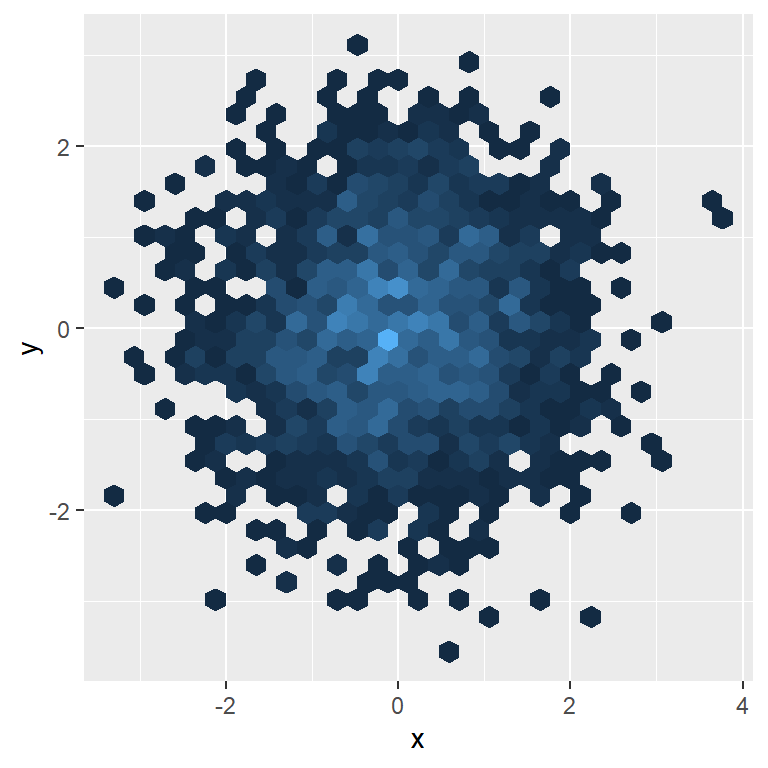# Hexbin chart in ggplot2

## The `geom_hex` function

Given a numerical data frame you can create a hexbin chart in ggplot2 making use of `geom_hex`.

``````# install.packages("ggplot2")
library(ggplot2)

# Data
set.seed(1)
df <- data.frame(x = rnorm(2000), y = rnorm(2000))

ggplot(df, aes(x = x, y = y)) +
geom_hex()``````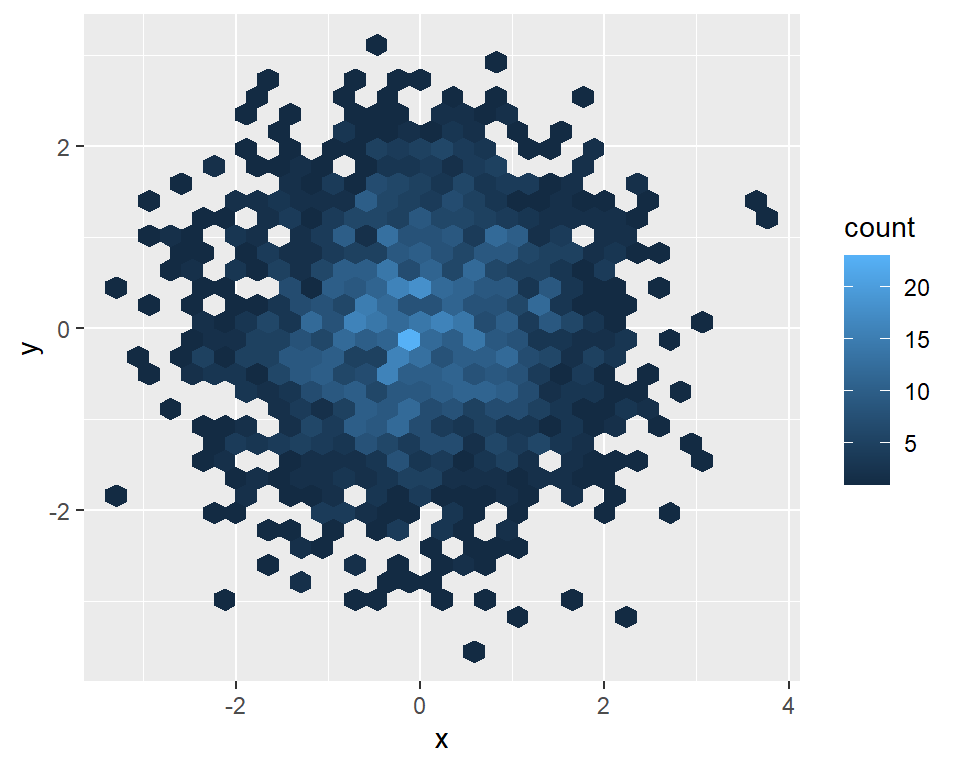Few bins

You can control de number of bins in both vertical and horizontal directions. Default value is 30.

``````# install.packages("ggplot2")
library(ggplot2)

# Data
set.seed(1)
df <- data.frame(x = rnorm(2000), y = rnorm(2000))

ggplot(df, aes(x = x, y = y)) +
geom_hex(bins = 15)``````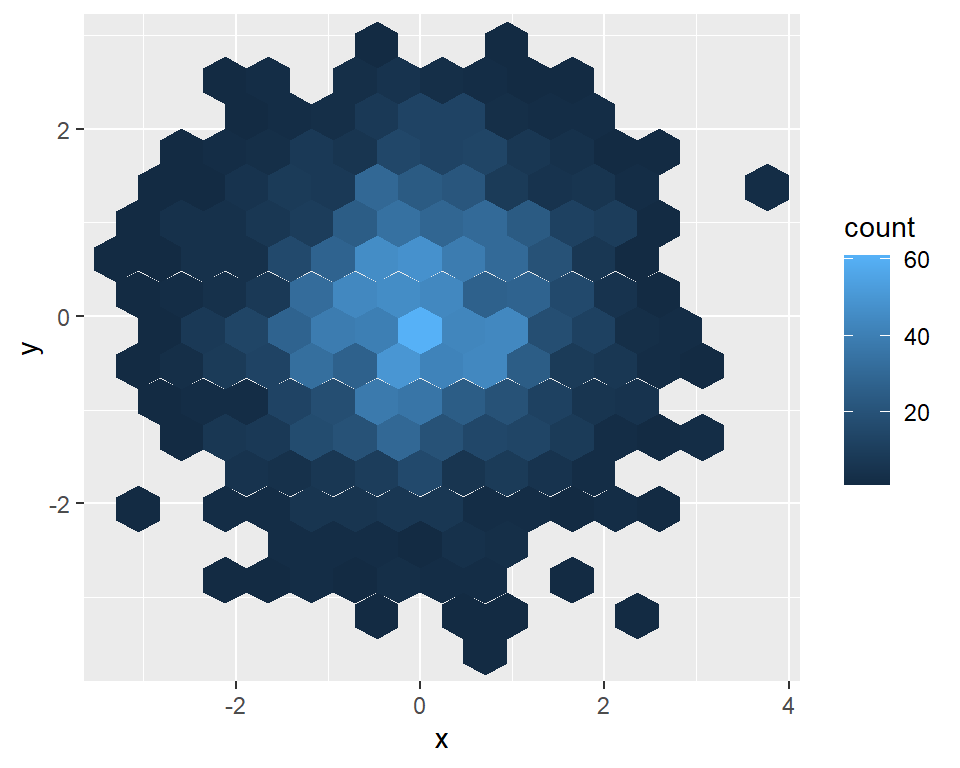Too many bins

Note that if you set too many bins the hexbin chart will look like a classical scatter plot.

``````# install.packages("ggplot2")
library(ggplot2)

# Data
set.seed(1)
df <- data.frame(x = rnorm(2000), y = rnorm(2000))

ggplot(df, aes(x = x, y = y)) +
geom_hex(bins = 60)``````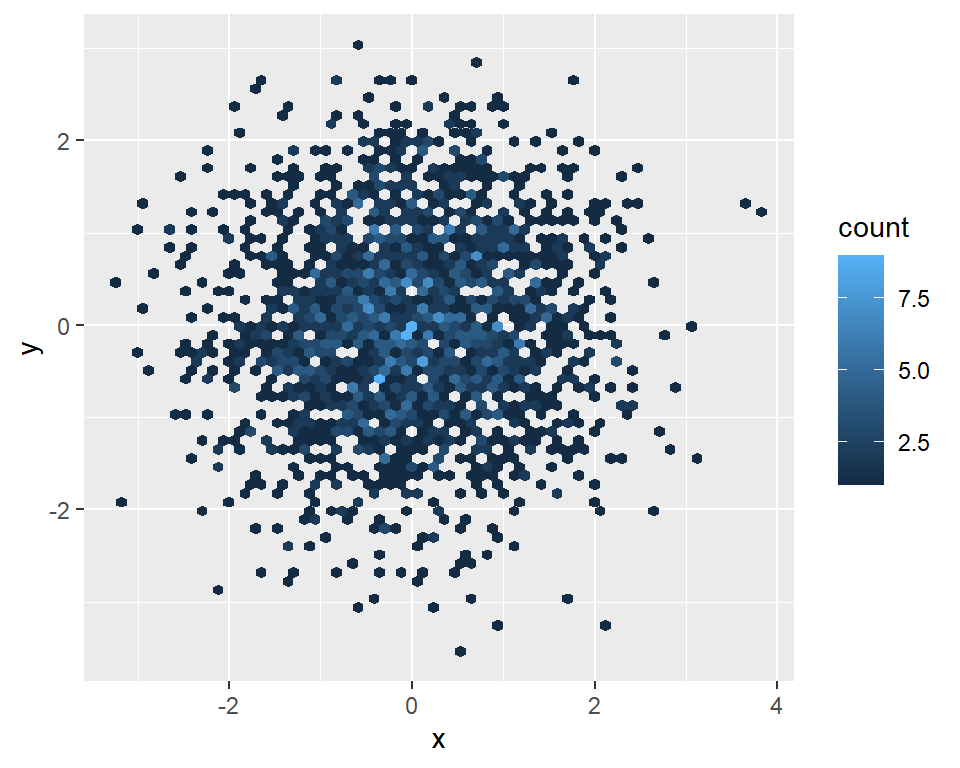## Color customization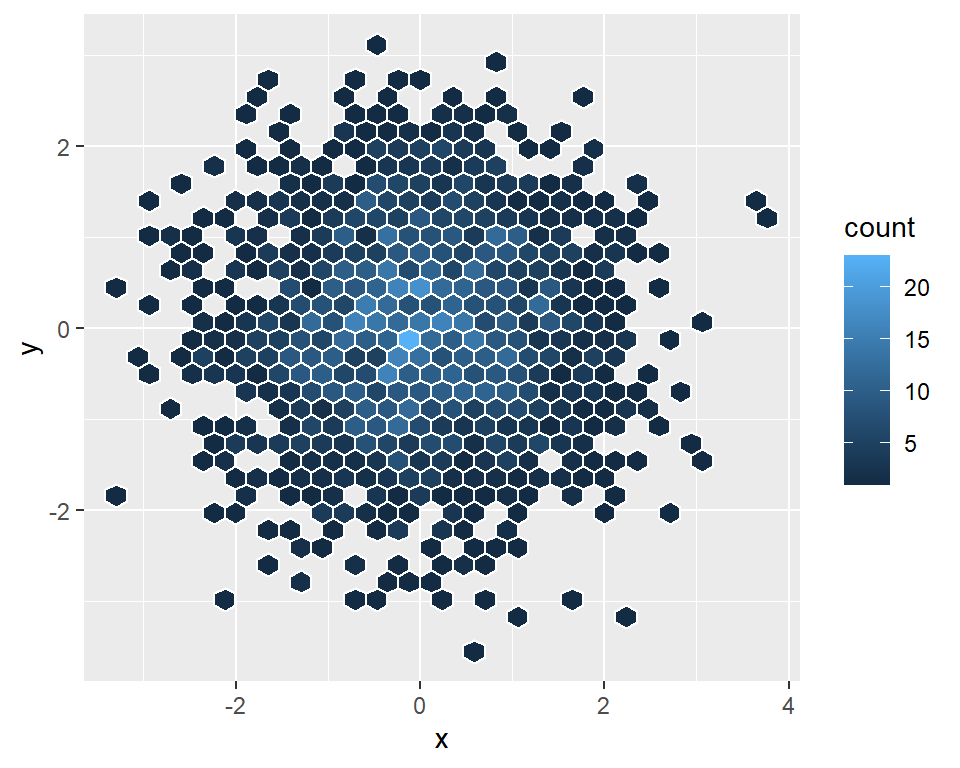Border color

The border for all hexagons can be customized with the `color` argument of the `geom_hex` function.

``````# install.packages("ggplot2")
library(ggplot2)

# Data
set.seed(1)
df <- data.frame(x = rnorm(2000), y = rnorm(2000))

ggplot(df, aes(x = x, y = y)) +
geom_hex(color = "white")``````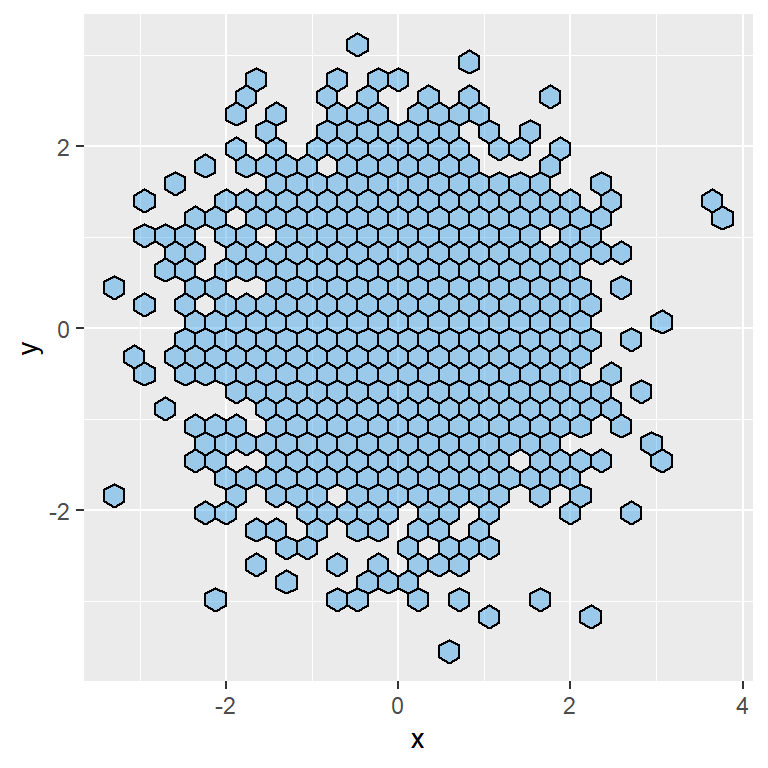Fill and transparency

You can also set a fill color for all the hexagons with `fill` and control the transparency of the colors with `alpha`.

``````# install.packages("ggplot2")
library(ggplot2)

# Data
set.seed(1)
df <- data.frame(x = rnorm(2000), y = rnorm(2000))

ggplot(df, aes(x = x, y = y)) +
geom_hex(color = 1, fill = 4, alpha = 0.4) ``````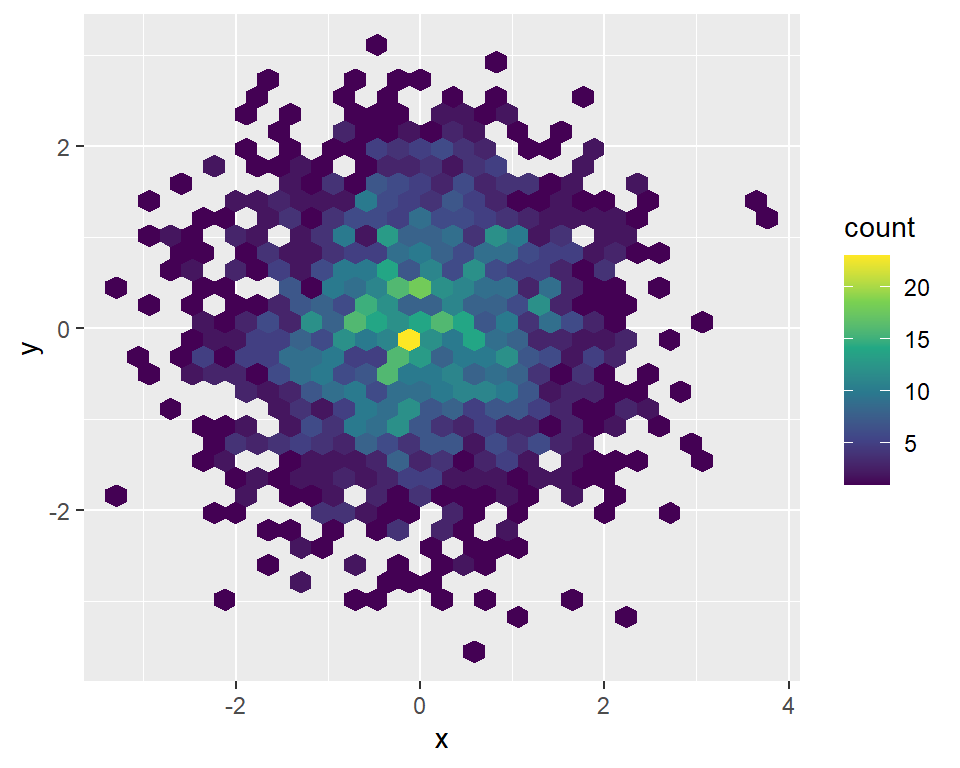Color palette

If you want to keep the gradient color palette representing values you can change the default colors with `scale_fill_viridis_c`, `scale_fill_gradient` or a similar function.

``````# install.packages("ggplot2")
library(ggplot2)

# Data
set.seed(1)
df <- data.frame(x = rnorm(2000), y = rnorm(2000))

ggplot(df, aes(x = x, y = y)) +
geom_hex() +
scale_fill_viridis_c()``````

## Legend customization

Width and height

When creating a hexbin chart a colour bar will be added to the chart by default. You can control the width and height of this bar with `barwidth` and `barheight`, as shown below.

``````# install.packages("ggplot2")
library(ggplot2)

# Data
set.seed(1)
df <- data.frame(x = rnorm(2000), y = rnorm(2000))

ggplot(df, aes(x = x, y = y)) +
geom_hex() +
guides(fill = guide_colourbar(barwidth = 0.7,
barheight = 15))``````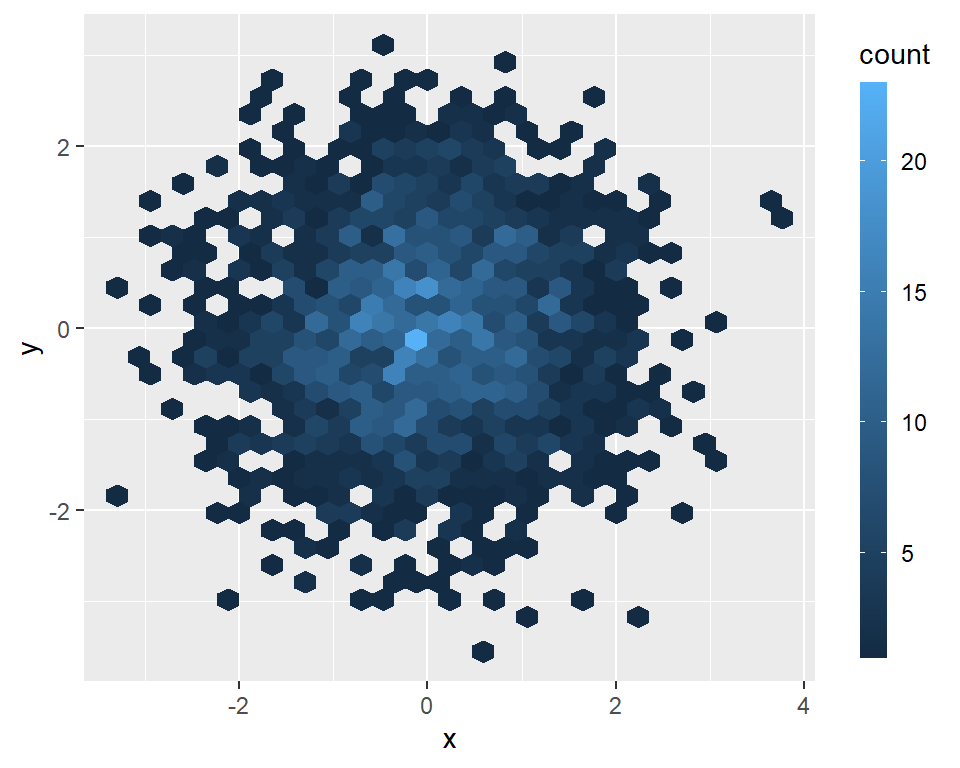Change the title

The default title of the legend is “count”. You can change it with the `title` argument of the `guide_colourbar` function as follows.

``````# install.packages("ggplot2")
library(ggplot2)

# Data
set.seed(1)
df <- data.frame(x = rnorm(2000), y = rnorm(2000))

ggplot(df, aes(x = x, y = y)) +
geom_hex() +
guides(fill = guide_colourbar(title = "Count"))``````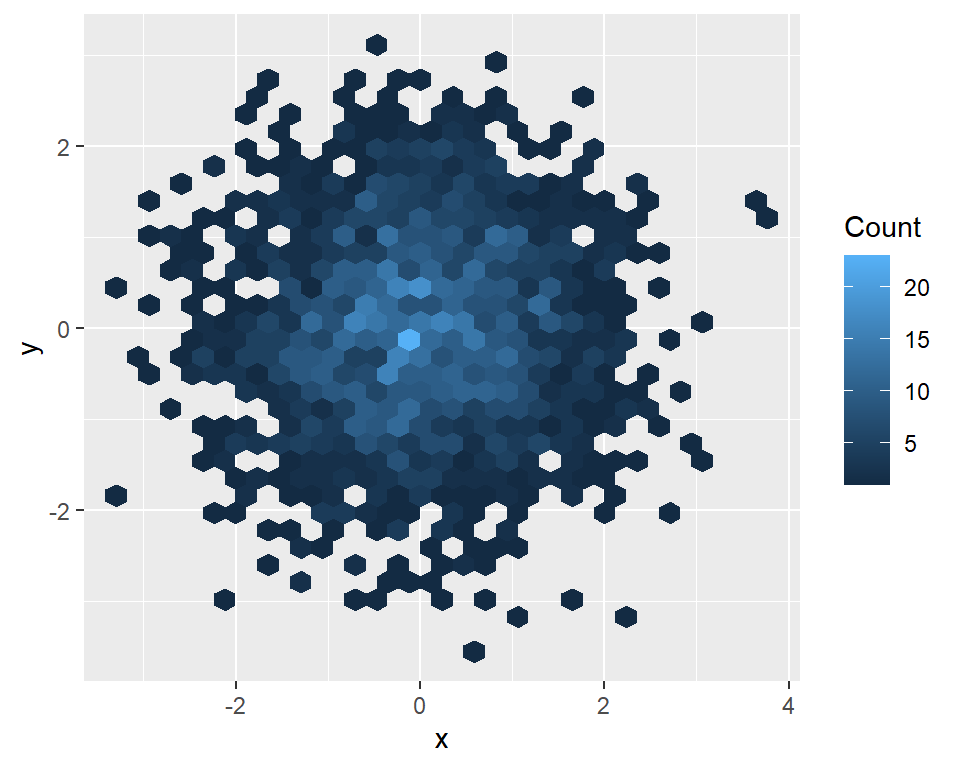Remove the labels and the ticks

Note that you can remove the ticks and/or the labels of the bar setting the `label` and `ticks` arguments to `FALSE`.

``````# install.packages("ggplot2")
library(ggplot2)

# Data
set.seed(1)
df <- data.frame(x = rnorm(2000), y = rnorm(2000))

ggplot(df, aes(x = x, y = y)) +
geom_hex() +
guides(fill = guide_colourbar(label = FALSE,
ticks = FALSE))``````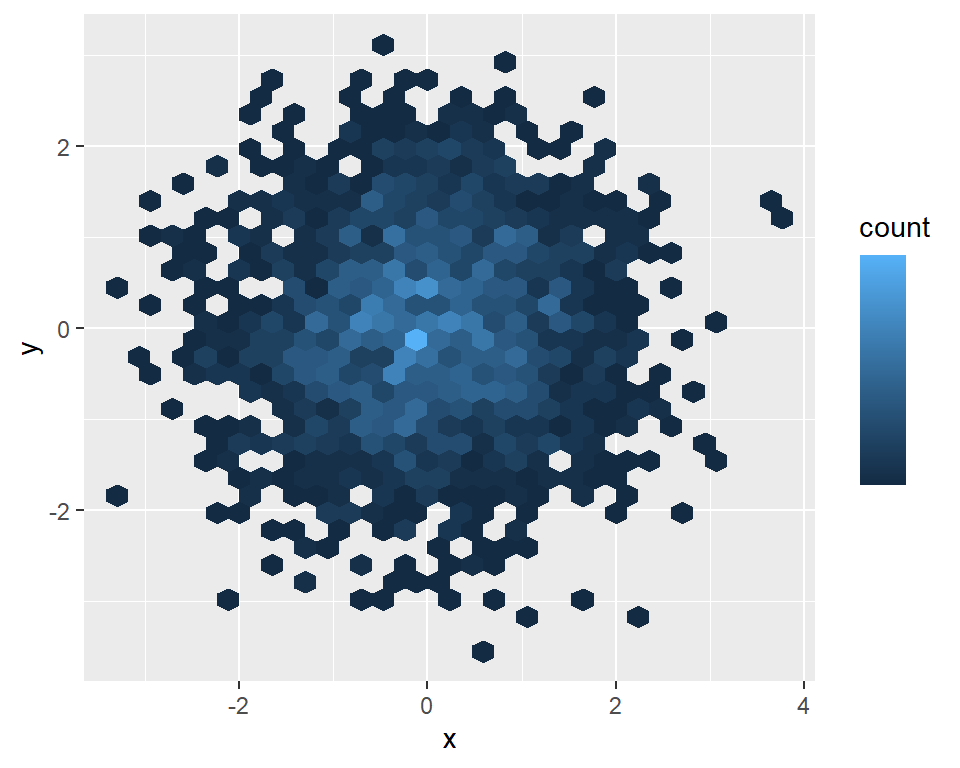Remove the legend

Finally, if you want to remove the legend you can set its position to `"none"`.

``````# install.packages("ggplot2")
library(ggplot2)

# Data
set.seed(1)
df <- data.frame(x = rnorm(2000), y = rnorm(2000))

ggplot(df, aes(x = x, y = y)) +
geom_hex() +
theme(legend.position = "none")``````